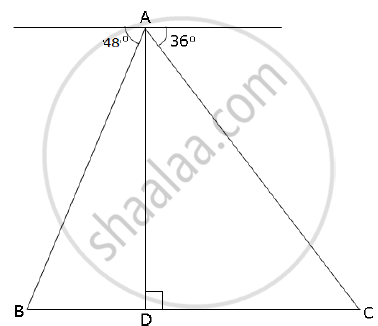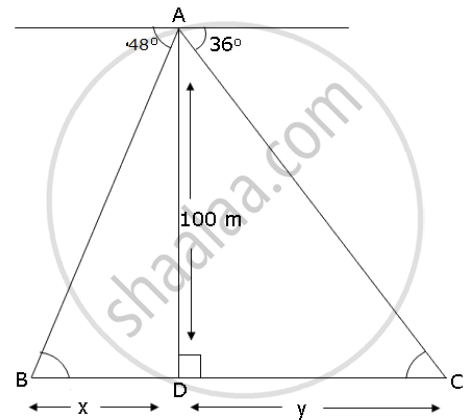# From the Top of a Lighthouse, 100 M High the Angles of Depression of Two Ships on Opposite Sides of It Are 48° and 36° Respectively. Find the Distance Between the Two Ships to the Nearest Metre. - Mathematics

From the top of a lighthouse, 100 m high the angles of depression of two ships on opposite sides of it are 48° and 36° respectively. Find the distance between the two ships to the nearest metre.#### Solution(AD)/(CD) = tan 36^@

=>100/y = tan 36^@

=> 100/tan 36^@ = 100/0.7265  => y = 137.638 m

100/x = tan 48^@ => x = 100/1.1106 = 90.04 m

∴ Distance between the ships = x + y

= 137.638 + 90.04 = 227.678 m

= 228 m (approx)

Concept: Heights and Distances - Solving 2-D Problems Involving Angles of Elevation and Depression Using Trigonometric Tables
Is there an error in this question or solution?